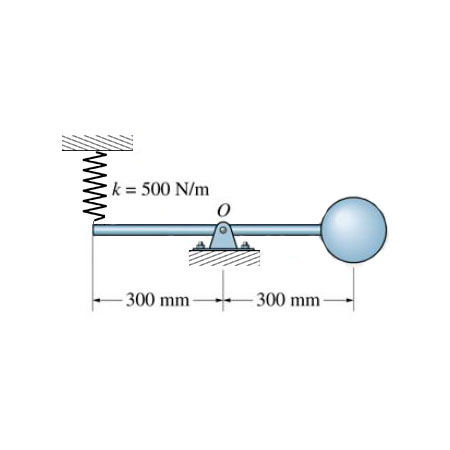# Determine the natural period of vibration of the 2 kg sphere. Neglect the mass of the rod and...

## Question:

Determine the natural period of vibration of the 2 kg sphere. Neglect the mass of the rod and than size the sphere.

Express your answer to three significant figures and include the appropriate units.## Spring-Mass system:

Time period of the natural vibration is given by:

{eq}\displaystyle T=2\Pi\sqrt{\frac{m}{k}} {/eq}

## Answer and Explanation: 1

Become a Study.com member to unlock this answer! Create your account

Given mass of sphere, m=2 kg and spring constant, k=500 N/m

Neglecting mass of the rod.

Here, the weight of ball pulls down the rod about the...

See full answer below.

#### Learn more about this topic:Practice Applying Spring Constant Formulas

from

Chapter 17 / Lesson 11
3.4K

In this lesson, you'll have the chance to practice using the spring constant formula. The lesson includes four problems of medium difficulty involving a variety of real-life applications.